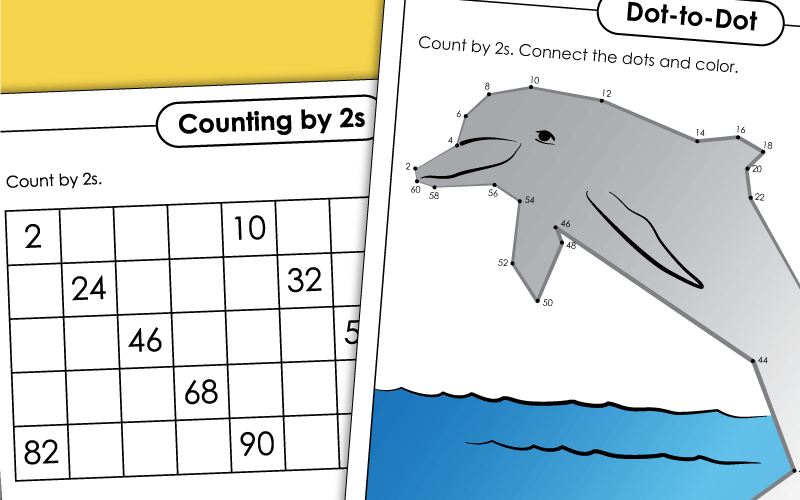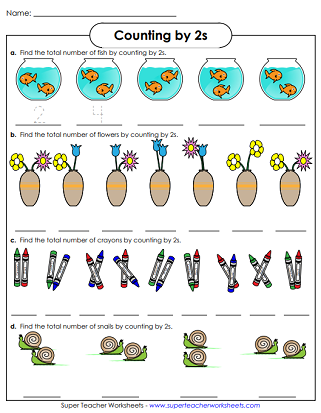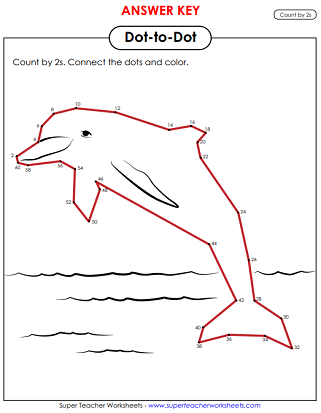# Skip Counting by 2

Learn to skip count by 2s.  Count 2, 4, 6, 8, 10, 12, and so on.Use this number line to count by 2s.  Complete the activity by filling in the boxes.  This number line ranges from 0 to 32.
Skip count by 2s, starting at 2 and ending at 20. Write the missing letters in the circles to help the mouse reach the cheese.
Count by 2s up to 100.  Fill in the empty boxes to complete the table.
Find the number of pictures in each set.  Count by twos.
The penguins on the ice floes are counting by twos.  Can you fill in the missing ones?
Count by 2s on this worksheet and fill in the missing numbers.  The page is decorated with two karate illustrations.
Skip count by 2s from 0 up to 40. Fill in the missing numbers on the number line.
Fill in the caterpillars as you skip count by twos.
Count by twos. Color every second number on the hundreds chart.
Print this skip-counting worksheet and answer the questions by counting by 2s.
Fill in the grid, counting by 2s. Answer the questions on the bottom of the page to practice skip counting by 2s.
The numbers on the rows of balloons count by two.  Complete the pattern.
Count by 2s and connect the dots to create a picture of a jumping dolphin.

More Skip Counting Worksheets

Count by threes, fours, tens, or fives.  Other skip counting worksheets can be found through this skip counting index page.

Counting Worksheets

Practice counting larger two, three, and four digit numbers.

Odd and Even Worksheets

These worksheets teach students the difference between odd and even numbers.

Counting Up to 20 (Very Basic)

These are our most basic counting worksheets.  Students practice counting up to twenty.

Hundreds Charts

We have all kinds of hundreds charts, hundreds chart games, and even 99s charts.

Color by Number Worksheets

These easy color-by-number worksheets are fun for kids of all ages.

## Pictures of WorksheetsMy Account
Site Information